# Maximum and Minimum Absolute Maximum or Minimum A

• Slides: 12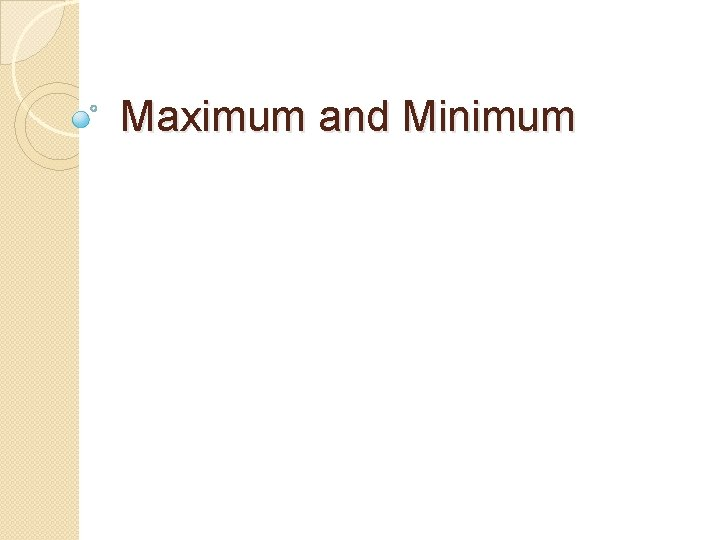Maximum and Minimum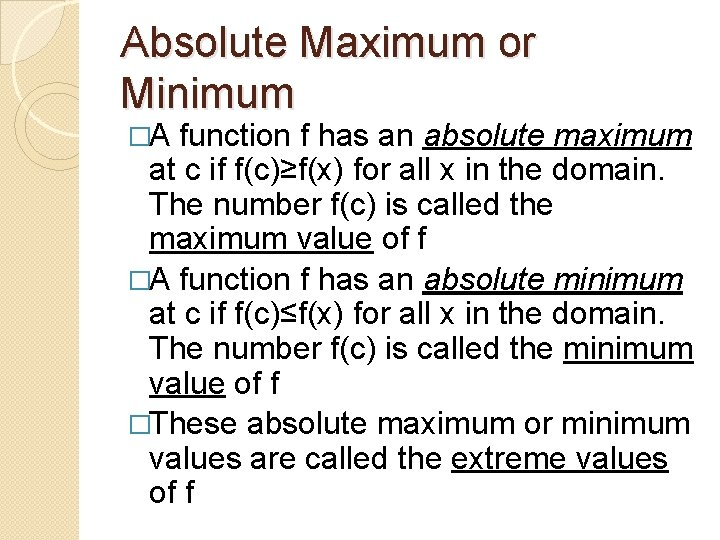Absolute Maximum or Minimum �A function f has an absolute maximum at c if f(c)≥f(x) for all x in the domain. The number f(c) is called the maximum value of f �A function f has an absolute minimum at c if f(c)≤f(x) for all x in the domain. The number f(c) is called the minimum value of f �These absolute maximum or minimum values are called the extreme values of f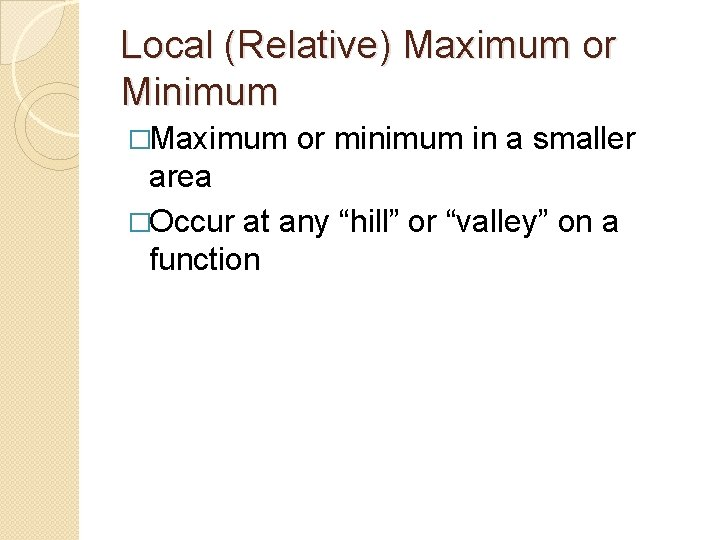Local (Relative) Maximum or Minimum �Maximum or minimum in a smaller area �Occur at any “hill” or “valley” on a function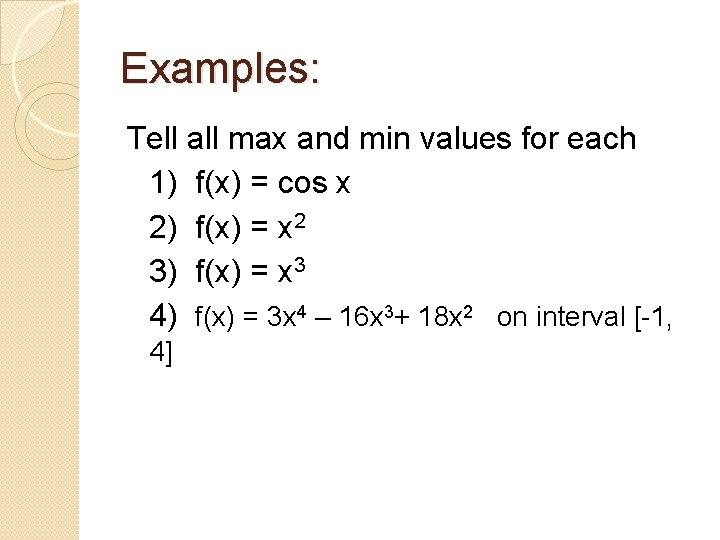Examples: Tell all max and min values for each 1) f(x) = cos x 2) f(x) = x 2 3) f(x) = x 3 4) f(x) = 3 x 4 – 16 x 3+ 18 x 2 on interval [-1, 4]Extreme Value Theorem �If f is continuous on a closed interval [ a, b], then f attains an absolute maximum f(c) and an absolute minimum f(d) at some number c and d in [a, b] - more than one extreme may exist - if f is not continuous, may not have an extreme - if the interval is not closed, there may not be an extremeFermat’s Theorm �If f has a local maximum or minimum at c, and if f’(c) exists, then f’(c) = 0 �Be careful, at every local max or min the tangent is horizontal, but not every horizontal tangent is a local max or min �Also may be a max or min at locations where f’ does not exist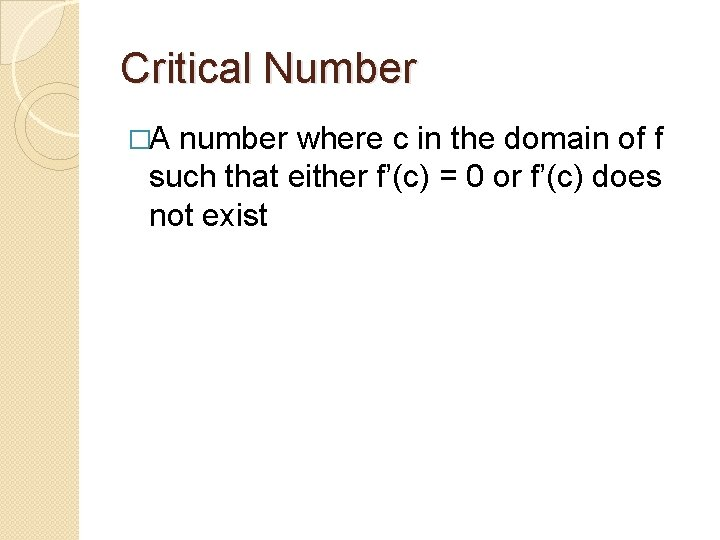Critical Number �A number where c in the domain of f such that either f’(c) = 0 or f’(c) does not exist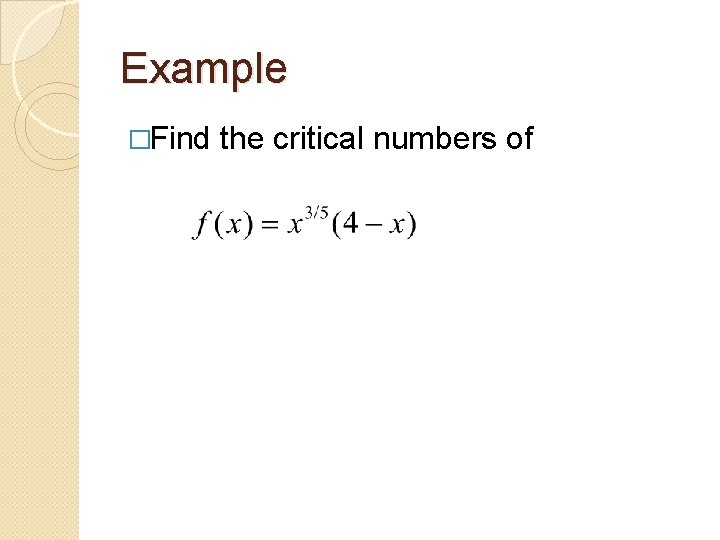Example �Find the critical numbers ofFinding Absolute Max and Min on a Closed Interval Find the critical numbers 2) Evaluate f at each critical number in the interval 3) Evaluate each endpoint of the interval 4) The least of these values is the minimum, the most is the maximum 1)Example Find the absolute maximum and minimum of on the interval [ -1/2, 4]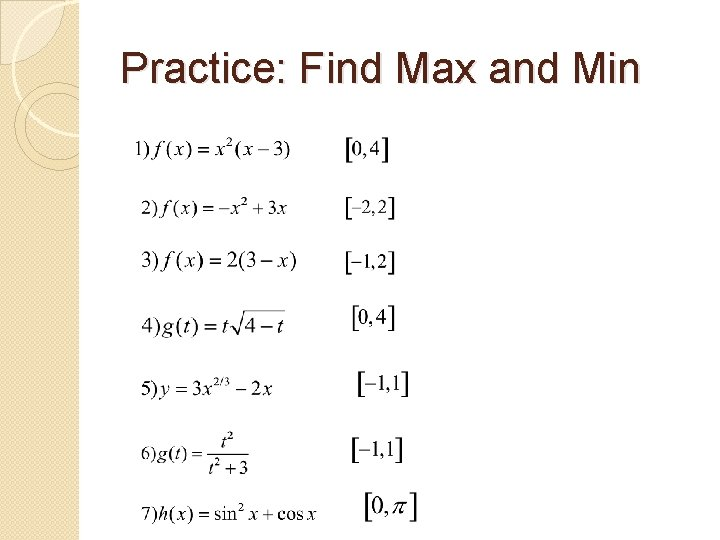Practice: Find Max and MinThe Hubble Space Telescope was deployed by the Space Shuttle. A model for the velocity of the shuttle during the mission from liftoff at t=0 to when the boosters were jettisoned at t=126 s is given by Use this model to estimate the maximum and minimum values of acceleration.